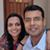2

We're looking for an AP-11 (Arithmetic progression of primes with 11 terms). So, we're looking for:

x_n = a + n.d  (for n = 0..k-1, k=11), such that all x_n are prime

Take any prime p, which doesn't divide a or d. Such a prime will divide every pth element of x_n. (Why? Think modular arithmetic. Since p doesn't divide d, successive x_n have to have different equivalents mod p, and we'll run out of such nonzero mods after at  most p elements of the sequence. As an exercise, try to express this proof mathematically).

Therefore, every such prime p, which is less than k, must divide d, or alternatively, if k is prime, we can have k=a, and all primes < k-1 dividing d.

So, for k=11 we can have

1.   a /= 11, and d is a multiple of 2*3*5*7*11  = 2310.
2.   a = 11  and d is a multiple of 2*3*5*7  = 210

Result follows evidently for case 1. For case 2 , a search over multiples should show that no such sequence exists. Is there an easier way for case 2?Mahesh Godavarti
0
Very nicely done.